# Draw it!

Draw two lines c, d that c || d. On line c, mark the points A, B. By point A, a lead perpendicular line to c. By point B, lead perpendicular line to c.

x =  0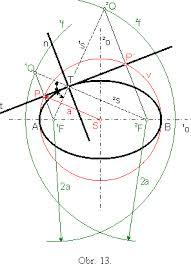Did you find an error or inaccuracy? Feel free to write us. Thank you!## Related math problems and questions:

• Straight lines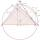Draw two lines c, d so that c || d. On line c mark points A, B, from point A start perpendicular to line c, from point B perpendicular to line c.
• Three points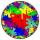Mark three points E, F and G in the plane not lie on one line. a) Draw a line segment FG b) Construct halfline (ray) EG c) Draw a line EF
• Rhombus constructionConstruct ABCD rhombus if its diagonal AC=9 cm and side AB = 6 cm. Inscribe a circle in it touching all sides...
• Parallels and one secant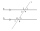There are two different parallel lines a, b, and a line c, that intersect the two parallel lines. Draw a circle that touches all lines at the same time.
• Square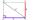Draw a square on the edge of a = 4 cm. Mark the center of symmetry S and all axes of symmetry. How many axes of symmetry does? Write down.
• Rhombus MATHConstruct a rhombus M A T H with diagonal MT=4cm, angle MAT=120°
• LinesHow many lines can be draw with 8 points, if three points lie on one line and the other any three points do not lie on the same line?
• Three points 2The three points A(3, 8), B(6, 2) and C(10, 2). The point D is such that the line DA is perpendicular to AB, and DC is parallel to AB. Calculate the coordinates of D.
• Hexagon - MOThe picture shows the ABCD square, the EFGD square and the HIJD rectangle. Points J and G lie on the side CD and is true |DJ|
• Find the 10Find the value of t if 2tx+5y-6=0 and 5x-4y+8=0 are perpendicular, parallel, what angle does each of the lines make with the x-axis, find the angle between the lines?
• Two chords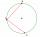There is a given circle k (center S, radius r). From point A which lies on circle k are starting two chords of length r. What angle does chords make? Draw and measure.
• Isosceles - isoscelesIt is given a triangle ABC with sides /AB/ = 3 cm /BC/ = 10 cm, and the angle ABC = 120°. Draw all points X such that true that BCX triangle is an isosceles and triangle ABX is isosceles with the base AB.
• RectangleDraw a rectangle with the sides a = 4 cm, b = 5 cm. Mark the center of symmetry S and all axes of symmetry. How many axes of symmetry has? Write down.
• Draw a trapezoidDraw a trapezoid if given a = 7 cm, b = 4 cm, c = 3.5 cm, diagonal AC = 5cm. Solve as a construction task.
• Line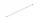How many parts of line divide 5 (different) points that lie on it?
• Angle between lines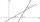Calculate the angle between these two lines: p: -8x +4y +5 =0 q: 10x +10y -7=0
• Draw a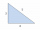Draw a triangle ABC, if you know: alpha = 60° side b = 4 cm side a = 10 cm